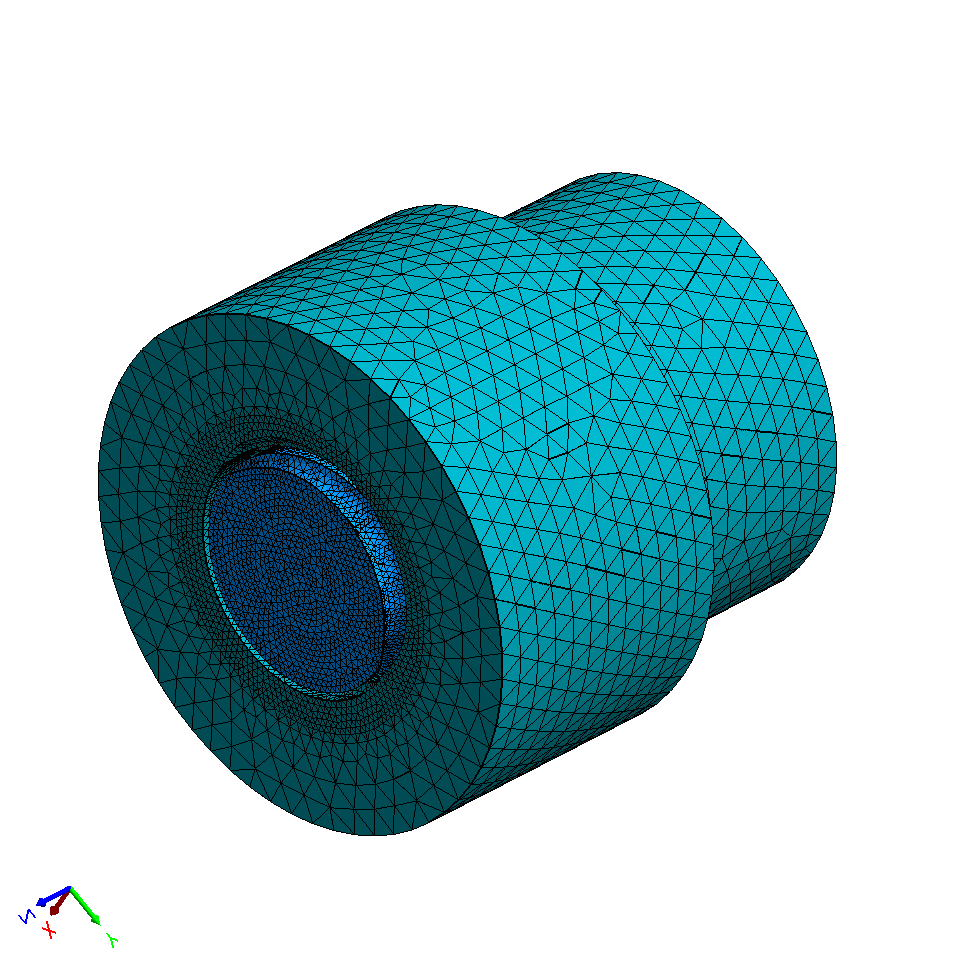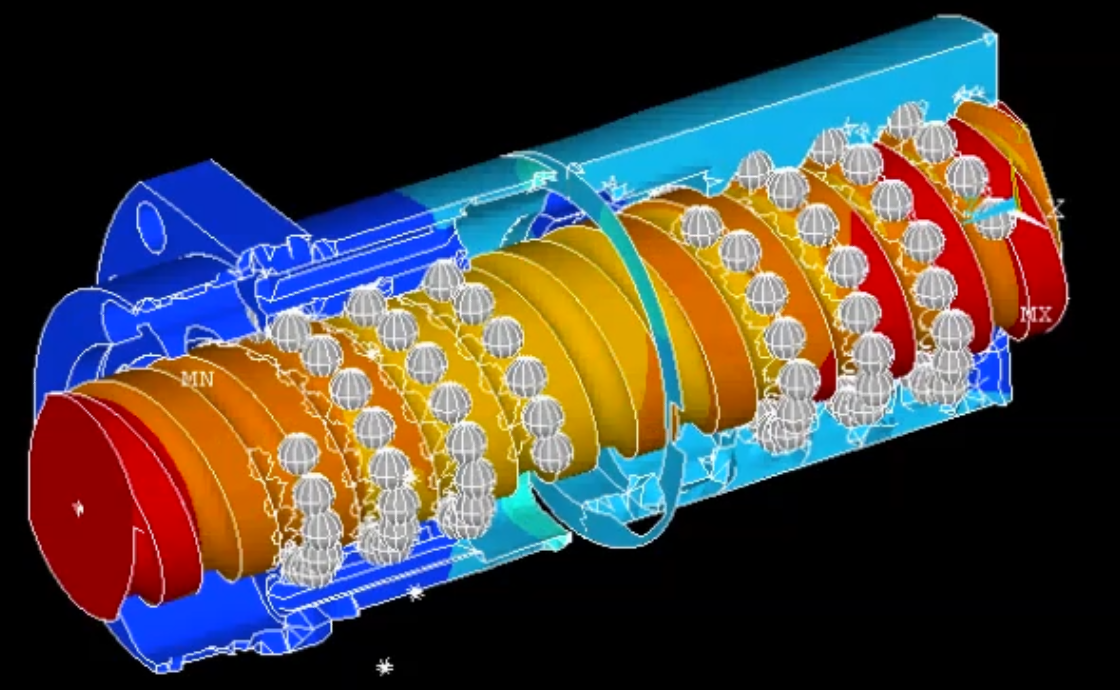###### Ball parameters
Nominal diameter (mm)
Pitch circle diameter (mm)
Elasticity modulus (GPa)
Poisson's ratio
Ball cage size (mm)
Maximal contact force (N)
###### Spindle parameters
Outer diameter (mm)
Bore diameter (mm)
Osculation
Contact angle (°)
Length (mm)
Elasticity modulus (GPa)
Poisson's ratio
Thermal dilatation coefficient (1/K)
###### Forces on left spindle end
Force in axial Z (N)
Suppressed rotation
###### Forces on right spindle end
Force in axial Z (N)
Suppressed rotation
Temperature of the spindle (C)
Temperature of the nut (C)
###### Nut parameters
Type of nut (single/double)
Pretension of double nut (N)
Pretension of double nut (µm)
Outer diameter (mm)
Inner diameter (mm)
Flange diameter (mm)
Flange thickness (mm)
Flange offset (mm)
Osculation
Contact angle (°)
Length of cylindrical nut (mm)
Length of flanged nut (mm)
Elasticity modulus (GPa)
Poisson's ratio
Thermal dilatation coefficient (1/K)
###### Ball circulations in cylindrical nut
Number of ball circulations
1st circulation
Min
Max
Middle
Balls
2nd circulation
Min
Max
Middle
Balls
3rd circulation
Min
Max
Middle
Balls
4th circulation
Min
Max
Middle
Balls
5th circulation
Min
Max
Middle
Balls
6th circulation
Min
Max
Middle
Kugeln
7th circulation
Min
Max
Middle
Kugeln
8th circulation
Min
Max
Middle
Kugeln
9th circulation
Min
Max
Middle
Kugeln
10th circulation
Min
Max
Middle
Kugeln
###### Ball circulations in flanged nut
Number of ball circulations
1st circulation
Min
Max
Middle
Balls
2nd circulation
Min
Max
Middle
Balls
3rd circulation
Min
Max
Middle
Balls
4th circulation
Min
Max
Middle
Balls
5th circulation
Min
Max
Middle
Balls
6th circulation
Min
Max
Middle
Kugeln
7th circulation
Min
Max
Middle
Kugeln
8th circulation
Min
Max
Middle
Kugeln
9th circulation
Min
Max
Middle
Kugeln
10th circulation
Min
Max
Middle
Kugeln
###### Nut flattening due to external ball circulations
Radial distance of the nut flattening (mm)
Length of the cylindrical nut flattening (mm)
Length of the flanged nut flattening (mm)

## Order options